TitleCollege Algebra
Tutorial 3: Scientific NotationAnswer/Discussion to 1a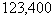*Decimal is at the end of the number   *Move decimal to create a number between 1 and 10

 How many decimal places did we end up moving? We started at the end of the number 123400 and moved it between the 1 and 2.  That looks like a move of 5 places. What direction did it move? Looks like we moved it to the left. So, our count is +5.Note how the number we started with is a bigger number than the one we are multiplying by in the scientific notation.  When that is the case, we will end up with a positive exponent.Answer/Discussion to 2a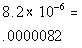*Move the decimal 6 to the leftAnswer/Discussion to 3a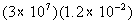*When mult. like bases you add your exponents: 7 + (-2) = 5 *Move the decimal 5 to the rightAnswer/Discussion to 3b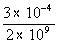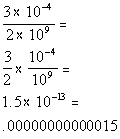*When div. like bases you subtract your exponents: -4 - 9 = -13   *Move the decimal 13 to the left

Last revised on Dec. 5, 2009 by Kim Seward.